xGolden ratioOverview

In mathematics
Mathematics
Mathematics is the study of quantity, space, structure, and change. Mathematicians seek out patterns and formulate new conjectures. Mathematicians resolve the truth or falsity of conjectures by mathematical proofs, which are arguments sufficient to convince other mathematicians of their validity...

and the art
Art
Art is the product or process of deliberately arranging items in a way that influences and affects one or more of the senses, emotions, and intellect....

s, two quantities are in the golden ratio if the ratio
Ratio
In mathematics, a ratio is a relationship between two numbers of the same kind , usually expressed as "a to b" or a:b, sometimes expressed arithmetically as a dimensionless quotient of the two which explicitly indicates how many times the first number contains the second In mathematics, a ratio is...

of the sum of the quantities to the larger quantity is equal to the ratio of the larger quantity to the smaller one. The golden ratio is an irrational
Irrational number
In mathematics, an irrational number is any real number that cannot be expressed as a ratio a/b, where a and b are integers, with b non-zero, and is therefore not a rational number....

mathematical constant
Mathematical constant
A mathematical constant is a special number, usually a real number, that is "significantly interesting in some way". Constants arise in many different areas of mathematics, with constants such as and occurring in such diverse contexts as geometry, number theory and calculus.What it means for a...

, approximately 1.61803398874989. Other names frequently used for the golden ratio are the golden section (Latin: sectio aurea) and golden mean.How do I find the golden ratio of a number say for instance the number 360?Encyclopedia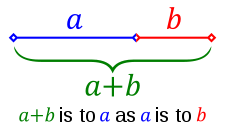In mathematics
Mathematics
Mathematics is the study of quantity, space, structure, and change. Mathematicians seek out patterns and formulate new conjectures. Mathematicians resolve the truth or falsity of conjectures by mathematical proofs, which are arguments sufficient to convince other mathematicians of their validity...

and the art
Art
Art is the product or process of deliberately arranging items in a way that influences and affects one or more of the senses, emotions, and intellect....

s, two quantities are in the golden ratio if the ratio
Ratio
In mathematics, a ratio is a relationship between two numbers of the same kind , usually expressed as "a to b" or a:b, sometimes expressed arithmetically as a dimensionless quotient of the two which explicitly indicates how many times the first number contains the second In mathematics, a ratio is...

of the sum of the quantities to the larger quantity is equal to the ratio of the larger quantity to the smaller one. The golden ratio is an irrational
Irrational number
In mathematics, an irrational number is any real number that cannot be expressed as a ratio a/b, where a and b are integers, with b non-zero, and is therefore not a rational number....

mathematical constant
Mathematical constant
A mathematical constant is a special number, usually a real number, that is "significantly interesting in some way". Constants arise in many different areas of mathematics, with constants such as and occurring in such diverse contexts as geometry, number theory and calculus.What it means for a...

, approximately 1.61803398874989. Other names frequently used for the golden ratio are the golden section (Latin: sectio aurea) and golden mean. Other terms encountered include extreme and mean ratio, medial section, divine proportion, divine section (Latin: sectio divina), golden proportion, golden cut, golden number, and mean of Phidias
Phidias
Phidias or the great Pheidias , was a Greek sculptor, painter and architect, who lived in the 5th century BC, and is commonly regarded as one of the greatest of all sculptors of Classical Greece: Phidias' Statue of Zeus at Olympia was one of the Seven Wonders of the Ancient World...

. In this article the golden ratio is denoted by the Greek
Greek alphabet
The Greek alphabet is the script that has been used to write the Greek language since at least 730 BC . The alphabet in its classical and modern form consists of 24 letters ordered in sequence from alpha to omega...

lowercase letter phi
Phi (letter)
Phi , pronounced or sometimes in English, and in modern Greek, is the 21st letter of the Greek alphabet. In modern Greek, it represents , a voiceless labiodental fricative. In Ancient Greek it represented , an aspirated voiceless bilabial plosive...

(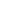), while its reciprocal,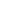or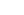, is denoted by the uppercase variant Phi
Phi (letter)
Phi , pronounced or sometimes in English, and in modern Greek, is the 21st letter of the Greek alphabet. In modern Greek, it represents , a voiceless labiodental fricative. In Ancient Greek it represented , an aspirated voiceless bilabial plosive...

(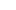).

The figure on the right illustrates the geometric relationship that defines this constant. Expressed algebraically: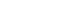This equation has one positive solution in the set of algebraic
Algebraic number
In mathematics, an algebraic number is a number that is a root of a non-zero polynomial in one variable with rational coefficients. Numbers such as π that are not algebraic are said to be transcendental; almost all real numbers are transcendental...

irrational number
Irrational number
In mathematics, an irrational number is any real number that cannot be expressed as a ratio a/b, where a and b are integers, with b non-zero, and is therefore not a rational number....

s: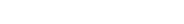.

At least since the Renaissance
Renaissance
The Renaissance was a cultural movement that spanned roughly the 14th to the 17th century, beginning in Italy in the Late Middle Ages and later spreading to the rest of Europe. The term is also used more loosely to refer to the historical era, but since the changes of the Renaissance were not...

, many artist
Artist
An artist is a person engaged in one or more of any of a broad spectrum of activities related to creating art, practicing the arts and/or demonstrating an art. The common usage in both everyday speech and academic discourse is a practitioner in the visual arts only...

s and architect
Architect
An architect is a person trained in the planning, design and oversight of the construction of buildings. To practice architecture means to offer or render services in connection with the design and construction of a building, or group of buildings and the space within the site surrounding the...

s have proportioned their works to approximate the golden ratio—especially in the form of the golden rectangle, in which the ratio of the longer side to the shorter is the golden ratio—believing this proportion to be aesthetically
Aesthetics
Aesthetics is a branch of philosophy dealing with the nature of beauty, art, and taste, and with the creation and appreciation of beauty. It is more scientifically defined as the study of sensory or sensori-emotional values, sometimes called judgments of sentiment and taste...

pleasing (see Applications and observations below). Mathematician
Mathematician
A mathematician is a person whose primary area of study is the field of mathematics. Mathematicians are concerned with quantity, structure, space, and change....

s have studied the golden ratio because of its unique and interesting properties. The golden ratio is also used in the analysis of financial markets, in strategies such as Fibonacci retracement
Fibonacci retracement
In finance, Fibonacci retracements is a method of technical analysis for determining support and resistance levels. They are named after their use of the Fibonacci sequence...

.## Calculation

 BinaryBinary numeral systemThe binary numeral system, or base-2 number system, represents numeric values using two symbols, 0 and 1. More specifically, the usual base-2 system is a positional notation with a radix of 2... 1.1001111000110111011… DecimalDecimalThe decimal numeral system has ten as its base. It is the numerical base most widely used by modern civilizations.... 1.6180339887498948482… HexadecimalHexadecimalIn mathematics and computer science, hexadecimal is a positional numeral system with a radix, or base, of 16. It uses sixteen distinct symbols, most often the symbols 0–9 to represent values zero to nine, and A, B, C, D, E, F to represent values ten to fifteen... 1.9E3779B97F4A7C15F39… Continued fractionContinued fractionIn mathematics, a continued fraction is an expression obtained through an iterative process of representing a number as the sum of its integer part and the reciprocal of another number, then writing this other number as the sum of its integer part and another reciprocal, and so on...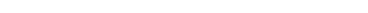Algebraic formAlgebraic numberIn mathematics, an algebraic number is a number that is a root of a non-zero polynomial in one variable with rational coefficients. Numbers such as π that are not algebraic are said to be transcendental; almost all real numbers are transcendental...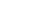Infinite series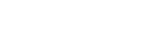Two quantities a and b are said to be in the golden ratio φ if: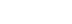.

One method for finding the value of φ is to start with the left fraction. Through simplifying the fraction and substituting in b/a = 1/φ,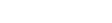,

it is shown that,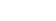Multiplying by φ gives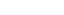which can be rearranged to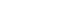.

Using the quadratic formula gives the only positive solution as,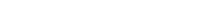.

## HistoryThe golden ratio has fascinated Western intellectuals of diverse interests for at least 2,400 years. According to Mario Livio
Mario Livio
Mario Livio is an astrophysicist and an author of works that popularize science and mathematics. He is currently an astronomer and head of public outreach at the Space Telescope Science Institute, which operates the Hubble Space Telescope...

:
Ancient Greek
Ancient Greece
Ancient Greece is a civilization belonging to a period of Greek history that lasted from the Archaic period of the 8th to 6th centuries BC to the end of antiquity. Immediately following this period was the beginning of the Early Middle Ages and the Byzantine era. Included in Ancient Greece is the...

mathematicians first studied what we now call the golden ratio because of its frequent appearance in geometry
Geometry
Geometry arose as the field of knowledge dealing with spatial relationships. Geometry was one of the two fields of pre-modern mathematics, the other being the study of numbers ....

. The division of a line into "extreme and mean ratio" (the golden section) is important in the geometry of regular pentagrams and pentagon
Pentagon
In geometry, a pentagon is any five-sided polygon. A pentagon may be simple or self-intersecting. The sum of the internal angles in a simple pentagon is 540°. A pentagram is an example of a self-intersecting pentagon.- Regular pentagons :In a regular pentagon, all sides are equal in length and...

s. The Greeks usually attributed discovery of this concept to Pythagoras
Pythagoras
Pythagoras of Samos was an Ionian Greek philosopher, mathematician, and founder of the religious movement called Pythagoreanism. Most of the information about Pythagoras was written down centuries after he lived, so very little reliable information is known about him...

or his followers
Pythagoreanism
Pythagoreanism was the system of esoteric and metaphysical beliefs held by Pythagoras and his followers, the Pythagoreans, who were considerably influenced by mathematics. Pythagoreanism originated in the 5th century BCE and greatly influenced Platonism...

. The regular pentagram, which has a regular pentagon inscribed within it, was the Pythagoreans' symbol.

Euclid
Euclid
Euclid , fl. 300 BC, also known as Euclid of Alexandria, was a Greek mathematician, often referred to as the "Father of Geometry". He was active in Alexandria during the reign of Ptolemy I...

's Elements
Euclid's Elements
Euclid's Elements is a mathematical and geometric treatise consisting of 13 books written by the Greek mathematician Euclid in Alexandria c. 300 BC. It is a collection of definitions, postulates , propositions , and mathematical proofs of the propositions...

(Greek
Greek language
Greek is an independent branch of the Indo-European family of languages. Native to the southern Balkans, it has the longest documented history of any Indo-European language, spanning 34 centuries of written records. Its writing system has been the Greek alphabet for the majority of its history;...

: ) provides the first known written definition of what is now called the golden ratio: "A straight line is said to have been cut in extreme and mean ratio when, as the whole line is to the greater segment, so is the greater to the less." Euclid explains a construction for cutting (sectioning) a line "in extreme and mean ratio", i.e. the golden ratio. Throughout the Elements, several propositions (theorem
Theorem
In mathematics, a theorem is a statement that has been proven on the basis of previously established statements, such as other theorems, and previously accepted statements, such as axioms...

s in modern terminology) and their proofs employ the golden ratio. Some of these propositions show that the golden ratio is an irrational number
Irrational number
In mathematics, an irrational number is any real number that cannot be expressed as a ratio a/b, where a and b are integers, with b non-zero, and is therefore not a rational number....

.

The name "extreme and mean ratio" was the principal term used from the 3rd century BC until about the 18th century.

The modern history of the golden ratio starts with Luca Pacioli
Luca Pacioli
Fra Luca Bartolomeo de Pacioli was an Italian mathematician, Franciscan friar, collaborator with Leonardo da Vinci, and seminal contributor to the field now known as accounting...

's De divina proportione
De divina proportione
De Divina Proportione is a famous book on mathematics written by Luca Pacioli around 1497 in Milan. Today only two versions of the original manuscript are believed still to exist...

of 1509, which captured the imagination of artists, architects, scientists, and mystics with the properties, mathematical and otherwise, of the golden ratio.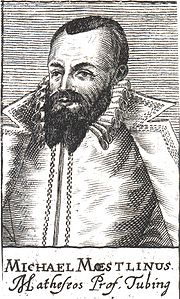The first known approximation of the (inverse) golden ratio by a decimal fraction, stated as "about 0.6180340," was written in 1597 by Michael Maestlin
Michael Maestlin
Michael Maestlin was a German astronomer and mathematician, known for being the mentor of Johannes Kepler.-Career:...

of the University of Tübingen in a letter to his former student Johannes Kepler
Johannes Kepler
Johannes Kepler was a German mathematician, astronomer and astrologer. A key figure in the 17th century scientific revolution, he is best known for his eponymous laws of planetary motion, codified by later astronomers, based on his works Astronomia nova, Harmonices Mundi, and Epitome of Copernican...

.

Since the twentieth century, the golden ratio has been represented by the Greek letter
Greek alphabet
The Greek alphabet is the script that has been used to write the Greek language since at least 730 BC . The alphabet in its classical and modern form consists of 24 letters ordered in sequence from alpha to omega...

Φ or φ (phi
Phi
Phi may refer to:In language:*Phi, the Greek letter Φ,φ, the symbol for voiceless bilabial fricativeIn mathematics:*The Golden ratio*Euler's totient function*A statistical measure of association reported with the chi-squared test...

, after Phidias
Phidias
Phidias or the great Pheidias , was a Greek sculptor, painter and architect, who lived in the 5th century BC, and is commonly regarded as one of the greatest of all sculptors of Classical Greece: Phidias' Statue of Zeus at Olympia was one of the Seven Wonders of the Ancient World...

, a sculptor who is said to have employed it) or less commonly by τ (tau
Tau
Tau is the 19th letter of the Greek alphabet. In the system of Greek numerals it has a value of 300.The name in English is pronounced , but in modern Greek it is...

, the first letter of the ancient Greek
Ancient Greek
Ancient Greek is the stage of the Greek language in the periods spanning the times c. 9th–6th centuries BC, , c. 5th–4th centuries BC , and the c. 3rd century BC – 6th century AD of ancient Greece and the ancient world; being predated in the 2nd millennium BC by Mycenaean Greek...

root τομή—meaning cut).

### Timeline

Timeline according to Priya Hemenway.
• Phidias
Phidias
Phidias or the great Pheidias , was a Greek sculptor, painter and architect, who lived in the 5th century BC, and is commonly regarded as one of the greatest of all sculptors of Classical Greece: Phidias' Statue of Zeus at Olympia was one of the Seven Wonders of the Ancient World...

Parthenon
The Parthenon is a temple on the Athenian Acropolis, Greece, dedicated to the Greek goddess Athena, whom the people of Athens considered their virgin patron. Its construction began in 447 BC when the Athenian Empire was at the height of its power. It was completed in 438 BC, although...

statues that seem to embody the golden ratio.
• Plato
Plato
Plato , was a Classical Greek philosopher, mathematician, student of Socrates, writer of philosophical dialogues, and founder of the Academy in Athens, the first institution of higher learning in the Western world. Along with his mentor, Socrates, and his student, Aristotle, Plato helped to lay the...

(427–347 BC), in his Timaeus
Timaeus (dialogue)
Timaeus is one of Plato's dialogues, mostly in the form of a long monologue given by the title character, written circa 360 BC. The work puts forward speculation on the nature of the physical world and human beings. It is followed by the dialogue Critias.Speakers of the dialogue are Socrates,...

, describes five possible regular solids (the Platonic solids: the tetrahedron
Tetrahedron
In geometry, a tetrahedron is a polyhedron composed of four triangular faces, three of which meet at each vertex. A regular tetrahedron is one in which the four triangles are regular, or "equilateral", and is one of the Platonic solids...

, cube
Cube
In geometry, a cube is a three-dimensional solid object bounded by six square faces, facets or sides, with three meeting at each vertex. The cube can also be called a regular hexahedron and is one of the five Platonic solids. It is a special kind of square prism, of rectangular parallelepiped and...

, octahedron
Octahedron
In geometry, an octahedron is a polyhedron with eight faces. A regular octahedron is a Platonic solid composed of eight equilateral triangles, four of which meet at each vertex....

, dodecahedron and icosahedron
Icosahedron
In geometry, an icosahedron is a regular polyhedron with 20 identical equilateral triangular faces, 30 edges and 12 vertices. It is one of the five Platonic solids....

), some of which are related to the golden ratio.
• Euclid
Euclid
Euclid , fl. 300 BC, also known as Euclid of Alexandria, was a Greek mathematician, often referred to as the "Father of Geometry". He was active in Alexandria during the reign of Ptolemy I...

(c. 325–c. 265 BC), in his Elements
Euclid's Elements
Euclid's Elements is a mathematical and geometric treatise consisting of 13 books written by the Greek mathematician Euclid in Alexandria c. 300 BC. It is a collection of definitions, postulates , propositions , and mathematical proofs of the propositions...

, gave the first recorded definition of the golden ratio, which he called, as translated into English, "extreme and mean ratio" (Greek: ἄκρος καὶ μέσος λόγος).
• Fibonacci
Fibonacci
Leonardo Pisano Bigollo also known as Leonardo of Pisa, Leonardo Pisano, Leonardo Bonacci, Leonardo Fibonacci, or, most commonly, simply Fibonacci, was an Italian mathematician, considered by some "the most talented western mathematician of the Middle Ages."Fibonacci is best known to the modern...

(1170–1250) mentioned the numerical series
Sequence
In mathematics, a sequence is an ordered list of objects . Like a set, it contains members , and the number of terms is called the length of the sequence. Unlike a set, order matters, and exactly the same elements can appear multiple times at different positions in the sequence...

now named after him in his Liber Abaci
Liber Abaci
Liber Abaci is a historic book on arithmetic by Leonardo of Pisa, known later by his nickname Fibonacci...

; the ratio of sequential elements of the Fibonacci sequence
Fibonacci number
In mathematics, the Fibonacci numbers are the numbers in the following integer sequence:0,\;1,\;1,\;2,\;3,\;5,\;8,\;13,\;21,\;34,\;55,\;89,\;144,\; \ldots\; ....

approaches the golden ratio asymptotically.
• Luca Pacioli
Luca Pacioli
Fra Luca Bartolomeo de Pacioli was an Italian mathematician, Franciscan friar, collaborator with Leonardo da Vinci, and seminal contributor to the field now known as accounting...

(1445–1517) defines the golden ratio as the "divine proportion" in his Divina Proportione.
• Johannes Kepler
Johannes Kepler
Johannes Kepler was a German mathematician, astronomer and astrologer. A key figure in the 17th century scientific revolution, he is best known for his eponymous laws of planetary motion, codified by later astronomers, based on his works Astronomia nova, Harmonices Mundi, and Epitome of Copernican...

(1571–1630) proves that the golden ratio is the limit of the ratio of consecutive Fibonacci numbers, and describes the golden ratio as a "precious jewel": "Geometry has two great treasures: one is the Theorem of Pythagoras
Pythagorean theorem
In mathematics, the Pythagorean theorem or Pythagoras' theorem is a relation in Euclidean geometry among the three sides of a right triangle...

, and the other the division of a line into extreme and mean ratio; the first we may compare to a measure of gold, the second we may name a precious jewel." These two treasures are combined in the Kepler triangle.
• Charles Bonnet
Charles Bonnet
Charles Bonnet , Swiss naturalist and philosophical writer, was born at Geneva, of a French family driven into Switzerland by the religious persecution in the 16th century.-Life and work:Bonnet's life was uneventful...

(1720–1793) points out that in the spiral phyllotaxis
Phyllotaxis
In botany, phyllotaxis or phyllotaxy is the arrangement of leaves on a plant stem .- Pattern structure :...

of plants going clockwise
Clockwise
Circular motion can occur in two possible directions. A clockwise motion is one that proceeds in the same direction as a clock's hands: from the top to the right, then down and then to the left, and back to the top...

and counter-clockwise were frequently two successive Fibonacci series.
• Martin Ohm
Martin Ohm
Martin Ohm was a German mathematician and a younger brother of physicist Georg Ohm. He earned his doctorate in 1811 at Friedrich-Alexander-University, Erlangen-Nuremberg where his advisor was Karl Christian von Langsdorf. Ohm was the first to fully develop the theory of the exponential ab when...

(1792–1872) is believed to be the first to use the term goldener Schnitt (golden section) to describe this ratio, in 1835.
• Édouard Lucas
Edouard Lucas
François Édouard Anatole Lucas was a French mathematician. Lucas is known for his study of the Fibonacci sequence. The related Lucas sequences and Lucas numbers are named after him.-Biography:...

(1842–1891) gives the numerical sequence now known as the Fibonacci sequence its present name.
• Mark Barr (20th century) suggests the Greek letter phi (φ), the initial letter of Greek sculptor Phidias's name, as a symbol
Symbol
A symbol is something which represents an idea, a physical entity or a process but is distinct from it. The purpose of a symbol is to communicate meaning. For example, a red octagon may be a symbol for "STOP". On a map, a picture of a tent might represent a campsite. Numerals are symbols for...

for the golden ratio.
• Roger Penrose
Roger Penrose
Sir Roger Penrose OM FRS is an English mathematical physicist and Emeritus Rouse Ball Professor of Mathematics at the Mathematical Institute, University of Oxford and Emeritus Fellow of Wadham College...

(b.1931) discovered a symmetrical pattern that uses the golden ratio in the field of aperiodic tiling
Aperiodic tiling
An aperiodic tiling is a tiling obtained from an aperiodic set of tiles. Properly speaking, aperiodicity is a property of particular sets of tiles; any given finite tiling is either periodic or non-periodic...

s, which led to new discoveries about quasicrystals.

### Aesthetics

De Divina Proportione, a three-volume work by Luca Pacioli
Luca Pacioli
Fra Luca Bartolomeo de Pacioli was an Italian mathematician, Franciscan friar, collaborator with Leonardo da Vinci, and seminal contributor to the field now known as accounting...

, was published in 1509. Pacioli, a Franciscan
Franciscan
Most Franciscans are members of Roman Catholic religious orders founded by Saint Francis of Assisi. Besides Roman Catholic communities, there are also Old Catholic, Anglican, Lutheran, ecumenical and Non-denominational Franciscan communities....

friar
Friar
A friar is a member of one of the mendicant orders.-Friars and monks:...

, was known mostly as a mathematician, but he was also trained and keenly interested in art. De Divina Proportione explored the mathematics of the golden ratio. Though it is often said that Pacioli advocated the golden ratio's application to yield pleasing, harmonious proportions, Livio points out that the interpretation has been traced to an error in 1799, and that Pacioli actually advocated the Vitruvian
Vitruvius
Marcus Vitruvius Pollio was a Roman writer, architect and engineer, active in the 1st century BC. He is best known as the author of the multi-volume work De Architectura ....

system of rational proportions. Pacioli also saw Catholic religious significance in the ratio, which led to his work's title. Containing illustrations of regular solids by Leonardo Da Vinci
Leonardo da Vinci
Leonardo di ser Piero da Vinci was an Italian Renaissance polymath: painter, sculptor, architect, musician, scientist, mathematician, engineer, inventor, anatomist, geologist, cartographer, botanist and writer whose genius, perhaps more than that of any other figure, epitomized the Renaissance...

, Pacioli's longtime friend and collaborator, De Divina Proportione was a major influence on generations of artists and architects alike.

### Architecture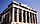The Parthenon's facade as well as elements of its facade and elsewhere are said by some to be circumscribed by golden rectangles. Other scholars deny that the Greeks had any aesthetic association with golden ratio. For example, Midhat J. Gazalé says, "It was not until Euclid, however, that the golden ratio's mathematical properties were studied. In the Elements (308 BC) the Greek mathematician merely regarded that number as an interesting irrational number, in connection with the middle and extreme ratios. Its occurrence in regular pentagons and decagons was duly observed, as well as in the dodecahedron (a regular polyhedron
Regular polyhedron
A regular polyhedron is a polyhedron whose faces are congruent regular polygons which are assembled in the same way around each vertex. A regular polyhedron is highly symmetrical, being all of edge-transitive, vertex-transitive and face-transitive - i.e. it is transitive on its flags...

whose twelve faces are regular pentagons). It is indeed exemplary that the great Euclid, contrary to generations of mystics who followed, would soberly treat that number for what it is, without attaching to it other than its factual properties." And Keith Devlin
Keith Devlin
Keith J. Devlin is a British mathematician and popular science writer. He has lived in the USA since 1987 and has dual American-British citizenship.- Biography :...

says, "Certainly, the oft repeated assertion that the Parthenon in Athens is based on the golden ratio is not supported by actual measurements. In fact, the entire story about the Greeks and golden ratio seems to be without foundation. The one thing we know for sure is that Euclid, in his famous textbook Elements, written around 300 BC, showed how to calculate its value." Near-contemporary sources like Vitruvius
Vitruvius
Marcus Vitruvius Pollio was a Roman writer, architect and engineer, active in the 1st century BC. He is best known as the author of the multi-volume work De Architectura ....

exclusively discuss proportions that can be expressed in whole numbers, i.e. commensurate as opposed to irrational proportions.

A geometrical analysis of the Great Mosque of Kairouan
Mosque of Oqba
The Mosque of Uqba , also known as the Great Mosque of Kairouan , is one of the most important mosques in Tunisia, situated in the UNESCO World Heritage town of Kairouan....

reveals a consistent application of the golden ratio throughout the design, according to Boussora and Mazouz. It is found in the overall proportion of the plan and in the dimensioning of the prayer space, the court, and the minaret
Minaret
A minaret مناره , sometimes مئذنه) is a distinctive architectural feature of Islamic mosques, generally a tall spire with an onion-shaped or conical crown, usually either free standing or taller than any associated support structure. The basic form of a minaret includes a base, shaft, and gallery....

. Boussora and Mazouz also examined earlier archaeological theories about the mosque, and demonstrate the geometric constructions based on the golden ratio by applying these constructions to the plan of the mosque to test their hypothesis.

The Swiss architect
Architect
An architect is a person trained in the planning, design and oversight of the construction of buildings. To practice architecture means to offer or render services in connection with the design and construction of a building, or group of buildings and the space within the site surrounding the...

Le Corbusier
Le Corbusier
Charles-Édouard Jeanneret, better known as Le Corbusier , was a Swiss-born French architect, designer, urbanist, writer and painter, famous for being one of the pioneers of what now is called modern architecture. He was born in Switzerland and became a French citizen in 1930...

, famous for his contributions to the modern
Modernism
Modernism, in its broadest definition, is modern thought, character, or practice. More specifically, the term describes the modernist movement, its set of cultural tendencies and array of associated cultural movements, originally arising from wide-scale and far-reaching changes to Western society...

international style
International style (architecture)
The International style is a major architectural style that emerged in the 1920s and 1930s, the formative decades of Modern architecture. The term originated from the name of a book by Henry-Russell Hitchcock and Philip Johnson, The International Style...

, centered his design philosophy on systems of harmony and proportion. Le Corbusier's faith in the mathematical order of the universe was closely bound to the golden ratio and the Fibonacci series, which he described as "rhythms apparent to the eye and clear in their relations with one another. And these rhythms are at the very root of human activities. They resound in man by an organic inevitability, the same fine inevitability which causes the tracing out of the Golden Section by children, old men, savages and the learned."

Le Corbusier explicitly used the golden ratio in his Modulor
Modulor
The Modulor is an anthropometric scale of proportions devised by the Swiss-born French architect Le Corbusier .It was developed as a visual bridge between two incompatible scales, the Imperial system and the Metric system...

system for the scale
Scale (ratio)
The scale ratio of some sort of model which represents an original proportionally is the ratio of a linear dimension of the model to the same dimension of the original. Examples include a 3-dimensional scale model of a building or the scale drawings of the elevations or plans of a building. In such...

of architectural proportion
Proportion (architecture)
Proportion is the relation between elements and a whole.-Architectural proportions:In architecture the whole is not just a building but the set and setting of the site. The things that make a building and its site "well shaped" include the orientation of the site and the buildings on it to the...

. He saw this system as a continuation of the long tradition of Vitruvius
Vitruvius
Marcus Vitruvius Pollio was a Roman writer, architect and engineer, active in the 1st century BC. He is best known as the author of the multi-volume work De Architectura ....

, Leonardo da Vinci's "Vitruvian Man
Vitruvian Man
The Vitruvian Man is a world-renowned drawing created by Leonardo da Vinci circa 1487. It is accompanied by notes based on the work of the famed architect, Vitruvius. The drawing, which is in pen and ink on paper, depicts a male figure in two superimposed positions with his arms and legs apart and...

", the work of Leon Battista Alberti, and others who used the proportions of the human body to improve the appearance and function of architecture
Architecture
Architecture is both the process and product of planning, designing and construction. Architectural works, in the material form of buildings, are often perceived as cultural and political symbols and as works of art...

. In addition to the golden ratio, Le Corbusier based the system on human measurements
Anthropometry
Anthropometry refers to the measurement of the human individual...

, Fibonacci numbers, and the double unit. He took suggestion of the golden ratio in human proportions to an extreme: he sectioned his model human body's height at the navel with the two sections in golden ratio, then subdivided those sections in golden ratio at the knees and throat; he used these golden ratio proportions in the Modulor
Modulor
The Modulor is an anthropometric scale of proportions devised by the Swiss-born French architect Le Corbusier .It was developed as a visual bridge between two incompatible scales, the Imperial system and the Metric system...

system. Le Corbusier's 1927 Villa Stein in Garches
Garches
Garches is a commune in the western suburbs of Paris, France. It is located from the centre of Paris.Garches has remained largely residential, but is also the location of the Hôpital Raymond Poincaré , which specialises in traumatology, road accidents and physiotherapy.-19 January Monument:The...

exemplified the Modulor system's application. The villa's rectangular ground plan, elevation, and inner structure closely approximate golden rectangles.

Another Swiss architect, Mario Botta
Mario Botta
Mario Botta is a Swiss architect. He studied at the Liceo Artistico in Milan and the IUAV in Venice. His ideas were influenced by Le Corbusier, Carlo Scarpa, Louis Kahn. He opened his own practice in 1970 in Lugano.-Career:...

, bases many of his designs on geometric figures. Several private houses he designed in Switzerland are composed of squares and circles, cubes and cylinders. In a house he designed in Origlio
Origlio
Origlio is a municipality in the district of Lugano in the canton of Ticino in Switzerland.-History:Origlio is first mentioned in 1335 as Orellio....

, the golden ratio is the proportion between the central section and the side sections of the house.

In a recent book, author Jason Elliot speculated that the golden ratio was used by the designers of the Naqsh-e Jahan Square and the adjacent Lotfollah mosque.

### Painting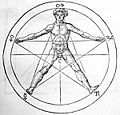The 16th-century philosopher Heinrich Agrippa drew a man over a pentagram inside a circle, implying a relationship to the golden ratio.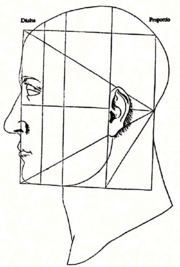Leonardo da Vinci
Leonardo da Vinci
Leonardo di ser Piero da Vinci was an Italian Renaissance polymath: painter, sculptor, architect, musician, scientist, mathematician, engineer, inventor, anatomist, geologist, cartographer, botanist and writer whose genius, perhaps more than that of any other figure, epitomized the Renaissance...

's illustrations of polyhedra in De divina proportione
De divina proportione
De Divina Proportione is a famous book on mathematics written by Luca Pacioli around 1497 in Milan. Today only two versions of the original manuscript are believed still to exist...

(On the Divine Proportion) and his views that some bodily proportions exhibit the golden ratio have led some scholars to speculate that he incorporated the golden ratio in his paintings. But the suggestion that his Mona Lisa
Mona Lisa
Mona Lisa is a portrait by the Italian artist Leonardo da Vinci. It is a painting in oil on a poplar panel, completed circa 1503–1519...

, for example, employs golden ratio proportions, is not supported by anything in Leonardo's own writings.

Salvador Domènec Felip Jacint Dalí i Domènech, Marquis de Púbol , commonly known as Salvador Dalí , was a prominent Spanish Catalan surrealist painter born in Figueres,Spain....

, influenced by the works of Matila Ghyka, explicitly used the golden ratio in his masterpiece, The Sacrament of the Last Supper
The Sacrament of the Last Supper
Completed in 1955 after nine months of work, Salvador Dalí’s painting The Sacrament of the Last Supper has remained one of his most popular compositions. Since its arrival at the National Gallery of Art in Washington, D.C...

. The dimensions of the canvas are a golden rectangle. A huge dodecahedron, in perspective so that edges appear in golden ratio to one another, is suspended above and behind Jesus and dominates the composition.

Mondrian
Piet Mondrian
Pieter Cornelis "Piet" Mondriaan, after 1906 Mondrian , was a Dutch painter.He was an important contributor to the De Stijl art movement and group, which was founded by Theo van Doesburg. He evolved a non-representational form which he termed Neo-Plasticism...

has been said to have used the golden section extensively in his geometrical paintings, though other experts (including critic Yve-Alain Bois
Yve-Alain Bois
Yve-Alain Bois is an historian and critic of modern art. Yve-Alain Bois was born on April 16, 1952 in Constantine, Algeria.-Education:...

) have disputed this claim.

A statistical study on 565 works of art of different great painters, performed in 1999, found that these artists had not used the golden ratio in the size of their canvases. The study concluded that the average ratio of the two sides of the paintings studied is 1.34, with averages for individual artists ranging from 1.04 (Goya) to 1.46 (Bellini). On the other hand, Pablo Tosto listed over 350 works by well-known artists, including more than 100 which have canvasses with golden rectangle and root-5 proportions, and others with proportions like root-2, 3, 4, and 6.

### Book design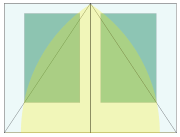According to Jan Tschichold
Jan Tschichold
Jan Tschichold was a typographer, book designer, teacher and writer.-Life:Tschichold was the son of a provincial signwriter, and he was trained in calligraphy...

,

There was a time when deviations from the truly beautiful page proportions 2:3, 1:√3, and the Golden Section were rare. Many books produced between 1550 and 1770 show these proportions exactly, to within half a millimeter.

### Perceptual studies

Studies by psychologists, starting with Fechner
Fechner
Fechner is a surname of:* Christian Fechner , a French film producer and screenwriter* Gustav Fechner , a German experimental psychologist* Harry Fechner , a German football defender...

, have been devised to test the idea that the golden ratio plays a role in human perception of beauty
Beauty
Beauty is a characteristic of a person, animal, place, object, or idea that provides a perceptual experience of pleasure, meaning, or satisfaction. Beauty is studied as part of aesthetics, sociology, social psychology, and culture...

. While Fechner found a preference for rectangle ratios centered on the golden ratio, later attempts to carefully test such a hypothesis have been, at best, inconclusive.

### Music

James Tenney
James Tenney
James Tenney was an American composer and influential music theorist.-Biography:Tenney was born in Silver City, New Mexico, and grew up in Arizona and Colorado. He attended the University of Denver, the Juilliard School of Music, Bennington College and the University of Illinois...

reconceived his piece For Ann (rising)
For Ann (rising)
For Ann is a piece of electronic music created by James Tenney in 1969.Tenney is the author of Meta Hodos, one of, if not the, earliest applications of gestalt theory and cognitive science to music, and later "Hierarchical temporal gestalt perception in music: a metric space model" with Larry...

, which consists of up to twelve computer-generated upwardly glissando
Glissando
In music, a glissando is a glide from one pitch to another. It is an Italianized musical term derived from the French glisser, to glide. In some contexts it is distinguished from the continuous portamento...

ing tones (see Shepard tone
Shepard tone
A Shepard tone, named after Roger Shepard, is a sound consisting of a superposition of sine waves separated by octaves. When played with the base pitch of the tone moving upward or downward, it is referred to as the Shepard scale. This creates the auditory illusion of a tone that continually...

), as having each tone start so it is the golden ratio (in between an equal tempered minor
Minor sixth
-Subminor sixth:In music, a subminor sixth or septimal sixth is an interval that is noticeably narrower than a minor sixth but noticeably wider than a diminished sixth.The sub-minor sixth is an interval of a 14:9 ratio or alternately 11:7....

and major sixth
Major sixth
In classical music from Western culture, a sixth is a musical interval encompassing six staff positions , and the major sixth is one of two commonly occurring sixths. It is qualified as major because it is the largest of the two...

) below the previous tone, so that the combination tones produced by all consecutive tones are a lower or higher pitch already, or soon to be, produced.

Ernő Lendvaï
Erno Lendvai
Ernő Lendvai was one of the first theorists to write on the appearance of the golden section and Fibonacci series and how these are implemented in Bartók's music...

analyzes Béla Bartók
Béla Bartók
Béla Viktor János Bartók was a Hungarian composer and pianist. He is considered one of the most important composers of the 20th century and is regarded, along with Liszt, as Hungary's greatest composer...

's works as being based on two opposing systems, that of the golden ratio and the acoustic scale
Acoustic scale
In music, the acoustic scale, overtone scale, Lydian dominant scale, or Lydian 7 scale, is a seven-note synthetic scale which, starting on C, contains the notes: C, D, E, F, G, A and B. This differs from the major scale in having a raised fourth and lowered seventh scale degree. It is the fourth...

, though other music scholars reject that analysis. In Bartok's Music for Strings, Percussion and Celesta
Music for Strings, Percussion and Celesta
Music for Strings, Percussion and Celesta, Sz. 106, BB 114 is one of the best-known compositions by the Hungarian composer Béla Bartók. Commissioned by Paul Sacher to celebrate the tenth anniversary of the Basel Chamber Orchestra, the score is dated September 7, 1936...

the xylophone progression occurs at the intervals 1:2:3:5:8:5:3:2:1. French composer Erik Satie
Erik Satie
Éric Alfred Leslie Satie was a French composer and pianist. Satie was a colourful figure in the early 20th century Parisian avant-garde...

used the golden ratio in several of his pieces, including Sonneries de la Rose+Croix.

The golden ratio is also apparent in the organization of the sections in the music of Debussy's Reflets dans l'eau
Reflets dans l'eau
Claude Debussy's piece Reflets dans l'eau is the first of three pieces for the piano from his first volume of Images, which are frequently performed separately. It was written in 1905...

(Reflections in Water)
, from Images (1st series, 1905), in which "the sequence of keys is marked out by the intervals 34, 21, 13 and 8, and the main climax sits at the phi position."

The musicologist Roy Howat has observed that the formal boundaries of La Mer
La Mer (Debussy)
La mer, trois esquisses symphoniques pour orchestre , or simply La mer , is an orchestral composition by the French composer Claude Debussy. It was started in 1903 in France and completed in 1905 on the English Channel coast in Eastbourne...

correspond exactly to the golden section. Trezise finds the intrinsic evidence "remarkable," but cautions that no written or reported evidence suggests that Debussy consciously sought such proportions.
Also, many works of Chopin, mainly Etudes (studies) and Nocturnes, are formally based on the golden ratio. This results in the biggest climax of both musical expression and technical difficulty after about 2/3 of the piece.

The mathematician Michael Schneider analysed the waveform of the Amen break
Amen break
The Amen break is a brief drum solo performed in 1969 by Gregory Cylvester "G. C." Coleman in the song "Amen, Brother" performed by the 1960s funk and soul outfit The Winstons...

and found that the peaks are spaced at intervals in the golden ratio.

Pearl Drums
Pearl Drums
Founded in 1952, the is a multinational corporation based in Japan with a wide range of products, predominately percussion instruments.-History:Pearl was founded by Katsumi Yanagisawa, who began manufacturing music stands in Sumida, Tokyo on April 2, 1946...

positions the air vents on its Masters Premium models based on the golden ratio. The company claims that this arrangement improves bass response and has applied for a patent
Patent
A patent is a form of intellectual property. It consists of a set of exclusive rights granted by a sovereign state to an inventor or their assignee for a limited period of time in exchange for the public disclosure of an invention....

on this innovation.

In the opinion of author Leon Harkleroad, "Some of the most misguided attempts to link music and mathematics have involved Fibonacci numbers and the related golden ratio."

### Industrial design

Some sources claim that the golden ratio is commonly used in everyday design, for example in the shapes of postcards, playing cards, posters, wide-screen televisions, photographs, and light switch plates.

### Nature

Adolf Zeising was a German psychologist, whose main interests were mathematics and philosophy.Among his discoveries, Zeising found the golden ratio expressed in the arrangement of branches along the stems of plants and of veins in leaves...

, whose main interests were mathematics and philosophy, found the golden ratio expressed in the arrangement of branches along the stems of plants and of veins in leaves. He extended his research to the skeletons of animals and the branchings of their veins and nerves, to the proportions of chemical compounds and the geometry of crystals, even to the use of proportion in artistic endeavors. In these phenomena he saw the golden ratio operating as a universal law. In connection with his scheme for golden-ratio-based human body proportions, Zeising wrote in 1854 of a universal law "in which is contained the ground-principle of all formative striving for beauty and completeness in the realms of both nature and art, and which permeates, as a paramount spiritual ideal, all structures, forms and proportions, whether cosmic or individual, organic or inorganic, acoustic or optical; which finds its fullest realization, however, in the human form."

In 2003, Volkmar Weiss
Volkmar Weiss
Volkmar Weiss is a German scientist and writer, primarily interested in the field of IQ research. He is mostly known for his controversial thesis that biological intelligence is fixed to social class and for co-founding the German Social Union.He was previously a member of the German Christian...

and Harald Weiss analyzed psychometric data and theoretical considerations and concluded that the golden ratio underlies the clock cycle of brain waves. In 2008 this was empirically confirmed by a group of neurobiologists.

In 2010, the journal Science reported that the golden ratio is present at the atomic scale in the magnetic resonance of spins in cobalt niobate crystals.

Several researchers have proposed connections between the golden ratio and human genome
Human genome
The human genome is the genome of Homo sapiens, which is stored on 23 chromosome pairs plus the small mitochondrial DNA. 22 of the 23 chromosomes are autosomal chromosome pairs, while the remaining pair is sex-determining...

DNA
DNA
Deoxyribonucleic acid is a nucleic acid that contains the genetic instructions used in the development and functioning of all known living organisms . The DNA segments that carry this genetic information are called genes, but other DNA sequences have structural purposes, or are involved in...

.

However, some have argued that many of the apparent manifestations of the golden mean in nature, especially in regard to animal dimensions, are in fact fictitious.

### Finance

The golden ratio and related numbers are used in the financial markets. It is used in trading algorithms, applications and strategies. Some typical forms include: the Fibonacci fan, the Fibonacci arc, Fibonacci retracement
Fibonacci retracement
In finance, Fibonacci retracements is a method of technical analysis for determining support and resistance levels. They are named after their use of the Fibonacci sequence...

and the Fibonacci time extension.

### Golden ratio conjugate

The negative root of the quadratic equation for φ (the "conjugate root") is.

The absolute value of this quantity (≈ 0.618) corresponds to the length ratio taken in reverse order (shorter segment length over longer segment length, b/a), and is sometimes referred to as the golden ratio conjugate. It is denoted here by the capital Phi (Φ):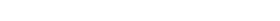.

Alternatively, Φ can be expressed as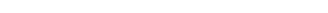.

This illustrates the unique property of the golden ratio among positive numbers, that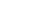.

or its inverse: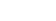.

This means 0.61803...:1 = 1:1.61803....

#### Contradiction from an expression in lowest terms

Recall that:
the whole is the longer part plus the shorter part;
the whole is to the longer part as the longer part is to the shorter part.

If we call the whole n and the longer part m, then the second statement above becomes
n is to m as m is to n − m,

or, algebraically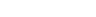To say that φ is rational means that φ is a fraction n/m where n and m are integers. We may take n/m to be in lowest terms and n and m to be positive. But if n/m is in lowest terms, then the identity labeled (*) above says m/(n − m) is in still lower terms. That is a contradiction that follows from the assumption that φ is rational.

#### Derivation from irrationality of √5

Another short proof—perhaps more commonly known—of the irrationality of the golden ratio makes use of the closure
Closure (mathematics)
In mathematics, a set is said to be closed under some operation if performance of that operation on members of the set always produces a unique member of the same set. For example, the real numbers are closed under subtraction, but the natural numbers are not: 3 and 8 are both natural numbers, but...

of rational numbers under addition and multiplication. Ifis rational, then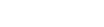is also rational, which is a contradiction if it is already known that the square root of a non-square
Square number
In mathematics, a square number, sometimes also called a perfect square, is an integer that is the square of an integer; in other words, it is the product of some integer with itself...

natural number
Natural number
In mathematics, the natural numbers are the ordinary whole numbers used for counting and ordering . These purposes are related to the linguistic notions of cardinal and ordinal numbers, respectively...

is irrational.

### Alternate forms

The formula φ = 1 + 1/φ can be expanded recursively to obtain a continued fraction
Continued fraction
In mathematics, a continued fraction is an expression obtained through an iterative process of representing a number as the sum of its integer part and the reciprocal of another number, then writing this other number as the sum of its integer part and another reciprocal, and so on...

for the golden ratio: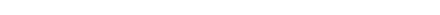and its reciprocal: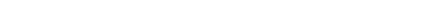The convergent
Convergent (continued fraction)
A convergent is one of a sequence of values obtained by evaluating successive truncations of a continued fraction The nth convergent is also known as the nth approximant of a continued fraction.-Representation of real numbers:...

s of these continued fractions (1/1, 2/1, 3/2, 5/3, 8/5, 13/8, …, or 1/1, 1/2, 2/3, 3/5, 5/8, 8/13, …) are ratios of successive Fibonacci numbers.

The equation φ2 = 1 + φ likewise produces the continued square root
Square root
In mathematics, a square root of a number x is a number r such that r2 = x, or, in other words, a number r whose square is x...

, or infinite surd, form: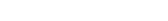.

An infinite series can be derived to express phi: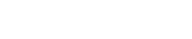Also: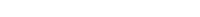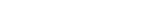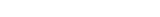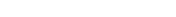These correspond to the fact that the length of the diagonal of a regular pentagon is φ times the length of its side, and similar relations in a pentagram
Pentagram
A pentagram is the shape of a five-pointed star drawn with five straight strokes...

.

### Geometry

The number φ turns up frequently in geometry
Geometry
Geometry arose as the field of knowledge dealing with spatial relationships. Geometry was one of the two fields of pre-modern mathematics, the other being the study of numbers ....

, particularly in figures with pentagonal symmetry
Symmetry
Symmetry generally conveys two primary meanings. The first is an imprecise sense of harmonious or aesthetically pleasing proportionality and balance; such that it reflects beauty or perfection...

.
The length of a regular pentagon
Pentagon
In geometry, a pentagon is any five-sided polygon. A pentagon may be simple or self-intersecting. The sum of the internal angles in a simple pentagon is 540°. A pentagram is an example of a self-intersecting pentagon.- Regular pentagons :In a regular pentagon, all sides are equal in length and...

's diagonal
Diagonal
A diagonal is a line joining two nonconsecutive vertices of a polygon or polyhedron. Informally, any sloping line is called diagonal. The word "diagonal" derives from the Greek διαγώνιος , from dia- and gonia ; it was used by both Strabo and Euclid to refer to a line connecting two vertices of a...

is φ times its side.
The vertices of a regular icosahedron
Icosahedron
In geometry, an icosahedron is a regular polyhedron with 20 identical equilateral triangular faces, 30 edges and 12 vertices. It is one of the five Platonic solids....

are those of three mutually orthogonal golden rectangles.

There is no known general algorithm
Algorithm
In mathematics and computer science, an algorithm is an effective method expressed as a finite list of well-defined instructions for calculating a function. Algorithms are used for calculation, data processing, and automated reasoning...

to arrange a given number of nodes evenly on a sphere, for any of several definitions of even distribution (see, for example, Thomson problem
Thomson problem
The Thomson problem is to determine the minimum energy configuration of N electrons on the surface of a sphere that repel each other with a force given by Coulomb's law. The physicist J. J...

). However, a useful approximation results from dividing the sphere into parallel bands of equal area
Area
Area is a quantity that expresses the extent of a two-dimensional surface or shape in the plane. Area can be understood as the amount of material with a given thickness that would be necessary to fashion a model of the shape, or the amount of paint necessary to cover the surface with a single coat...

and placing one node in each band at longitudes spaced by a golden section of the circle, i.e. 360°/φ 222.5°. This method was used to arrange the 1500 mirrors of the student-participatory satellite Starshine-3
STARSHINE
The STARSHINE series of three artificial satellites were student participatory missions sponsored by the United States Naval Research Laboratory ....

.

#### Dividing a line segment

A line segment
Line segment
In geometry, a line segment is a part of a line that is bounded by two end points, and contains every point on the line between its end points. Examples of line segments include the sides of a triangle or square. More generally, when the end points are both vertices of a polygon, the line segment...

can be divided according to the golden ratio with the following geometric construction:
• First, construct a line segment BC, perpendicular to the original line segment AB, passing through its endpoint B, and half the length of AB. Draw the hypotenuse AC.
• Draw a circle with center C and radius B. It intsersects the hypotenuse AC at point D.
• Draw a circle with center A and radius D. It intersects the original line segment AB at point S. This point divides the original segment AB in the golden ratio.

#### Golden triangle, pentagon and pentagram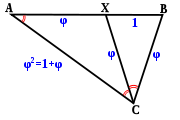##### Golden triangle

The golden triangle can be characterized as an isosceles triangle ABC with the property that bisecting
Bisection
In geometry, bisection is the division of something into two equal or congruent parts, usually by a line, which is then called a bisector. The most often considered types of bisectors are the segment bisector and the angle bisector In geometry, bisection is the division of something into two equal...

the angle C produces a new triangle
Triangle
A triangle is one of the basic shapes of geometry: a polygon with three corners or vertices and three sides or edges which are line segments. A triangle with vertices A, B, and C is denoted ....

CXB which is a similar triangle to the original.

If angle BCX = α, then XCA = α because of the bisection, and CAB = α because of the similar triangles; ABC = 2α from the original isosceles symmetry, and BXC = 2α by similarity. The angles in a triangle add up to 180°, so 5α = 180, giving α = 36°. So the angles of the golden triangle are thus 36°-72°-72°. The angles of the remaining obtuse isosceles triangle AXC (sometimes called the golden gnomon) are 36°-36°-108°.

Suppose XB has length 1, and we call BC length φ. Because of the isosceles triangles XC=XA and BC=XC, so these are also length φ. Length AC = AB, therefore equals φ+1. But triangle ABC is similar to triangle CXB, so AC/BC = BC/BX, and so AC also equals φ2. Thus φ2 = φ+1, confirming that φ is indeed the golden ratio.

Similarly, the ratio of the area of the larger triangle AXC to the smaller CXB is equal to φ, while the inverse
Inverse (mathematics)
In many contexts in mathematics the term inverse indicates the opposite of something. This word and its derivatives are used widely in mathematics, as illustrated below....

ratio is φ - 1.
##### Pentagon

In a regular pentagon the ratio between a side and a diagonal is(i.e. 1/φ), while intersecting diagonals section each other in the golden ratio.
##### Odom's construction

George Odom
George Phillips Odom, Jr
George Phillips Odom, Jr is an American artist and amateur geometer, who is primarily known for his work on the golden ratio .-Life and work:...

has given a remarkably simple construction for φ involving an equilateral triangle: if an equilateral triangle is inscribed in a circle and the line segment joining the midpoints of two sides is produced to intersect the circle in either of two points, then these three points are in golden proportion. This result is a straightforward consequence of the intersecting chords theorem and can be used to construct a regular pentagon, a construction that attracted the attention of the noted Canadian geometer H. S. M. Coxeter
Harold Scott MacDonald Coxeter
Harold Scott MacDonald "Donald" Coxeter, was a British-born Canadian geometer. Coxeter is regarded as one of the great geometers of the 20th century. He was born in London but spent most of his life in Canada....

who published it in Odom's name as a diagram in the American Mathematical Monthly
American Mathematical Monthly
The American Mathematical Monthly is a mathematical journal founded by Benjamin Finkel in 1894. It is currently published 10 times each year by the Mathematical Association of America....

accompanied by the single word "Behold!"
##### Pentagram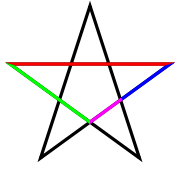The golden ratio plays an important role in the geometry of pentagram
Pentagram
A pentagram is the shape of a five-pointed star drawn with five straight strokes...

s. Each intersection of edges sections other edges in the golden ratio. Also, the ratio of the length of the shorter segment to the segment bounded by the two intersecting edges (a side of the pentagon in the pentagram's center) is φ, as the four-color illustration shows.

The pentagram includes ten isosceles triangles: five acute and five obtuse isosceles triangles. In all of them, the ratio of the longer side to the shorter side is φ. The acute triangles are golden triangles. The obtuse isosceles triangles are golden gnomons.
##### Ptolemy's theorem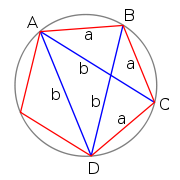The golden ratio properties of a regular pentagon can be confirmed by applying Ptolemy's theorem
Ptolemy's theorem
In Euclidean geometry, Ptolemy's theorem is a relation between the four sides and two diagonals of a cyclic quadrilateral . The theorem is named after the Greek astronomer and mathematician Ptolemy...

to the quadrilateral formed by removing one of its vertices. If the quadrilateral's long edge and diagonals are b, and short edges are a, then Ptolemy's theorem gives b2 = a2 + ab which yields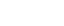.

#### Scalenity of triangles

Consider a triangle
Triangle
A triangle is one of the basic shapes of geometry: a polygon with three corners or vertices and three sides or edges which are line segments. A triangle with vertices A, B, and C is denoted ....

with sides of lengths a, b, and c in decreasing order. Define the "scalenity" of the triangle to be the smaller of the two ratios a/b and b/c. The scalenity is always less than φ and can be made as close as desired to φ.

#### Triangle whose sides form a geometric progression

If the side lengths of a triangle form a geometric progression
Geometric progression
In mathematics, a geometric progression, also known as a geometric sequence, is a sequence of numbers where each term after the first is found by multiplying the previous one by a fixed non-zero number called the common ratio. For example, the sequence 2, 6, 18, 54, ... is a geometric progression...

and are in the ratio 1 : r : r2, where r is the common ratio, then r must lie in the range φ−1 < r < φ, which is a consequence of the triangle inequality
Triangle inequality
In mathematics, the triangle inequality states that for any triangle, the sum of the lengths of any two sides must be greater than or equal to the length of the remaining side ....

(the sum of any two sides of a triangle must be strictly bigger than the length of the third side). If r = φ then the shorter two sides are 1 and φ but their sum is φ2, thus r < φ. A similar calculation shows that r> φ−1. A triangle whose sides are in the ratio 1 : √φ : φ is a right triangle (because 1 + φ = φ2) known as a Kepler triangle.

#### Golden triangle, rhombus, and rhombic triacontahedronA golden rhombus is a rhombus
Rhombus
In Euclidean geometry, a rhombus or rhomb is a convex quadrilateral whose four sides all have the same length. The rhombus is often called a diamond, after the diamonds suit in playing cards, or a lozenge, though the latter sometimes refers specifically to a rhombus with a 45° angle.Every...

whose diagonals are in the golden ratio. The rhombic triacontahedron
Rhombic triacontahedron
In geometry, the rhombic triacontahedron is a convex polyhedron with 30 rhombic faces. It is an Archimedean dual solid, or a Catalan solid. It is the polyhedral dual of the icosidodecahedron, and it is a zonohedron....

is a convex polytope
Convex polytope
A convex polytope is a special case of a polytope, having the additional property that it is also a convex set of points in the n-dimensional space Rn...

that has a very special property: all of its faces are golden rhombi. In the rhombic triacontahedron
Rhombic triacontahedron
In geometry, the rhombic triacontahedron is a convex polyhedron with 30 rhombic faces. It is an Archimedean dual solid, or a Catalan solid. It is the polyhedral dual of the icosidodecahedron, and it is a zonohedron....

the dihedral angle
Dihedral angle
In geometry, a dihedral or torsion angle is the angle between two planes.The dihedral angle of two planes can be seen by looking at the planes "edge on", i.e., along their line of intersection...

between any two adjacent rhombi is 144°, which is twice the isosceles angle of a golden triangle and four times its most acute angle.

### Relationship to Fibonacci sequence

The mathematics of the golden ratio and of the Fibonacci sequence
Fibonacci number
In mathematics, the Fibonacci numbers are the numbers in the following integer sequence:0,\;1,\;1,\;2,\;3,\;5,\;8,\;13,\;21,\;34,\;55,\;89,\;144,\; \ldots\; ....

are intimately interconnected. The Fibonacci sequence is:
0, 1, 1, 2, 3, 5, 8, 13, 21, 34, 55, 89, 144, 233, 377, 610, 987, …

The closed-form expression
Closed-form expression
In mathematics, an expression is said to be a closed-form expression if it can be expressed analytically in terms of a bounded number of certain "well-known" functions...

(known as Binet
Jacques Philippe Marie Binet
Jacques Philippe Marie Binet was a French mathematician, physicist and astronomer born in Rennes; he died in Paris, France, in 1856. He made significant contributions to number theory, and the mathematical foundations of matrix algebra which would later lead to important contributions by Cayley...

's formula, even though it was already known by Abraham de Moivre
Abraham de Moivre
Abraham de Moivre was a French mathematician famous for de Moivre's formula, which links complex numbers and trigonometry, and for his work on the normal distribution and probability theory. He was a friend of Isaac Newton, Edmund Halley, and James Stirling...

) for the Fibonacci sequence involves the golden ratio: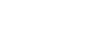.The golden ratio is the limit
Limit of a sequence
The limit of a sequence is, intuitively, the unique number or point L such that the terms of the sequence become arbitrarily close to L for "large" values of n...

of the ratios of successive terms of the Fibonacci sequence (or any Fibonacci-like sequence), as originally shown by Kepler: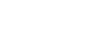Therefore, if a Fibonacci number is divided by its immediate predecessor in the sequence, the quotient approximates φ; e.g., 987/610 ≈ 1.6180327868852. These approximations are alternately lower and higher than φ, and converge on φ as the Fibonacci numbers increase, and: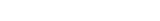.

More generally: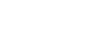where above, the ratios of consecutive terms of the Fibonacci sequence, is a case when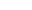.

Furthermore, the successive powers of φ obey the Fibonacci recurrence
Recurrence relation
In mathematics, a recurrence relation is an equation that recursively defines a sequence, once one or more initial terms are given: each further term of the sequence is defined as a function of the preceding terms....

: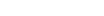.

This identity allows any polynomial in φ to be reduced to a linear expression. For example: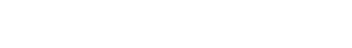However, this is no special property of φ, because polynomials in any solution x to a quadratic equation
In mathematics, a quadratic equation is a univariate polynomial equation of the second degree. A general quadratic equation can be written in the formax^2+bx+c=0,\,...

can be reduced in an analogous manner, by applying: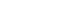.
for given coefficients a, b such that x satisfies the equation. Even more generally, any rational function
Rational function
In mathematics, a rational function is any function which can be written as the ratio of two polynomial functions. Neither the coefficients of the polynomials nor the values taken by the function are necessarily rational.-Definitions:...

(with rational coefficients) of the root of an irreducible nth-degree polynomial over the rationals can be reduced to a polynomial of degree n ‒ 1. Phrased in terms of field theory
Field theory (mathematics)
Field theory is a branch of mathematics which studies the properties of fields. A field is a mathematical entity for which addition, subtraction, multiplication and division are well-defined....

, if α is a root of an irreducible nth-degree polynomial, then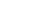has degree n over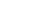, with basis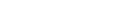.

### Symmetries

The golden ratio and inverse golden ratio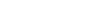have a set of symmetries that preserve and interrelate them. They are both preserved by the fractional linear transformations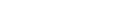– this fact corresponds to the identity and the definition quadratic equation.
Further, they are interchanged by the three maps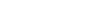– they are reciprocals, symmetric about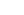, and (projectively) symmetric about 2.

More deeply, these maps form a subgroup of the modular group
Modular group
In mathematics, the modular group Γ is a fundamental object of study in number theory, geometry, algebra, and many other areas of advanced mathematics...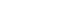isomorphic to the symmetric group
Symmetric group
In mathematics, the symmetric group Sn on a finite set of n symbols is the group whose elements are all the permutations of the n symbols, and whose group operation is the composition of such permutations, which are treated as bijective functions from the set of symbols to itself...

on 3 letters,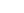corresponding to the stabilizer of the set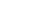of 3 standard points on the projective line
Projective line
In mathematics, a projective line is a one-dimensional projective space. The projective line over a field K, denoted P1, may be defined as the set of one-dimensional subspaces of the two-dimensional vector space K2 .For the generalisation to the projective line over an associative ring, see...

, and the symmetries correspond to the quotient map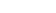– the subgroup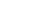consisting of the 3-cycles and the identity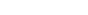fixes the two numbers, while the 2-cycles interchange these, thus realizing the map.

### Other properties

The golden ratio has the simplest expression (and slowest convergence) as a continued fraction expansion of any irrational number (see Alternate forms above). It is, for that reason, one of the worst cases of Lagrange's approximation theorem. This may be the reason angles close to the golden ratio often show up in phyllotaxis
Phyllotaxis
In botany, phyllotaxis or phyllotaxy is the arrangement of leaves on a plant stem .- Pattern structure :...

(the growth of plants).

The defining quadratic polynomial and the conjugate relationship lead to decimal values that have their fractional part in common with φ: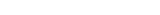.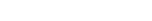.

The sequence of powers of φ contains these values 0.618…, 1.0, 1.618…, 2.618…; more generally,
any power of φ is equal to the sum of the two immediately preceding powers: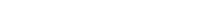.

As a result, one can easily decompose any power of φ into a multiple of φ and a constant. The multiple and the constant are always adjacent Fibonacci numbers. This leads to another property of the positive powers of φ:

If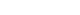, then: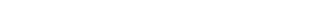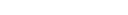When the golden ratio is used as the base of a numeral system
Numeral system
A numeral system is a writing system for expressing numbers, that is a mathematical notation for representing numbers of a given set, using graphemes or symbols in a consistent manner....

(see Golden ratio base
Golden ratio base
Golden ratio base is a non-integer positional numeral system that uses the golden ratio as its base. It is sometimes referred to as base-φ, golden mean base, phi-base, or, colloquially, phinary...

, sometimes dubbed phinary or φ-nary), every integer has a terminating representation, despite φ being irrational, but every fraction has a non-terminating representation.

The golden ratio is a fundamental unit
Fundamental unit (number theory)
In algebraic number theory, a fundamental unit is a generator for the torsion-free unit group of the ring of integers of a number field, when that group is infinite cyclic...

of the algebraic number field
Algebraic number field
In mathematics, an algebraic number field F is a finite field extension of the field of rational numbers Q...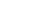and is a Pisot–Vijayaraghavan number. In the fieldwe have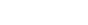, where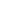is the-th Lucas number
Lucas number
The Lucas numbers are an integer sequence named after the mathematician François Édouard Anatole Lucas , who studied both that sequence and the closely related Fibonacci numbers...

.

The golden ratio also appears in hyperbolic geometry
Hyperbolic geometry
In mathematics, hyperbolic geometry is a non-Euclidean geometry, meaning that the parallel postulate of Euclidean geometry is replaced...

, as the maximum distance from a point on one side of an ideal triangle
Ideal triangle
In hyperbolic geometry an ideal triangle is a hyperbolic triangle whose three vertices all lie on the circle at infinity. In the hyperbolic metric, any two ideal triangles are congruent...

to the closer of the other two sides: this distance, the side length of the equilateral triangle formed by the points of tangency of a circle inscribed within the ideal triangle, is 4 ln φ.

### Decimal expansion

The golden ratio's decimal expansion can be calculated directly from the expression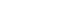with √5 ≈ 2.2360679774997896964. The square root of 5 can be calculated with the Babylonian method, starting with an initial estimate such as xφ = 2 and iterating
Iterative method
In computational mathematics, an iterative method is a mathematical procedure that generates a sequence of improving approximate solutions for a class of problems. A specific implementation of an iterative method, including the termination criteria, is an algorithm of the iterative method...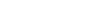for n = 1, 2, 3, …, until the difference between xn and xn−1 becomes zero, to the desired number of digits.

The Babylonian algorithm for √5 is equivalent to Newton's method
Newton's method
In numerical analysis, Newton's method , named after Isaac Newton and Joseph Raphson, is a method for finding successively better approximations to the roots of a real-valued function. The algorithm is first in the class of Householder's methods, succeeded by Halley's method...

for solving the equation x2 − 5 = 0. In its more general form, Newton's method can be applied directly to any algebraic equation, including the equation x2 − x − 1 = 0 that defines the golden ratio. This gives an iteration that converges to the golden ratio itself,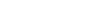for an appropriate initial estimate xφ such as xφ = 1. A slightly faster method is to rewrite the equation as x − 1 − 1/x = 0, in which case the Newton iteration becomes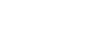These iterations all converge quadratically; that is, each step roughly doubles the number of correct digits. The golden ratio is therefore relatively easy to compute with arbitrary precision
Arbitrary-precision arithmetic
In computer science, arbitrary-precision arithmetic indicates that calculations are performed on numbers whose digits of precision are limited only by the available memory of the host system. This contrasts with the faster fixed-precision arithmetic found in most ALU hardware, which typically...

. The time needed to compute n digits of the golden ratio is proportional to the time needed to divide two n-digit numbers. This is considerably faster than known algorithms for the transcendental number
Transcendental number
In mathematics, a transcendental number is a number that is not algebraic—that is, it is not a root of a non-constant polynomial equation with rational coefficients. The most prominent examples of transcendental numbers are π and e...

s π
Pi
' is a mathematical constant that is the ratio of any circle's circumference to its diameter. is approximately equal to 3.14. Many formulae in mathematics, science, and engineering involve , which makes it one of the most important mathematical constants...

and e
E (mathematical constant)
The mathematical constant ' is the unique real number such that the value of the derivative of the function at the point is equal to 1. The function so defined is called the exponential function, and its inverse is the natural logarithm, or logarithm to base...

.

An easily programmed alternative using only integer arithmetic is to calculate two large consecutive Fibonacci numbers and divide them. The ratio of Fibonacci numbers F25001 and F25000, each over 5000 digits, yields over 10,000 significant digits of the golden ratio.

The golden ratio φ has been calculated to an accuracy of several millions of decimal digits . Alexis Irlande performed computations and verification of the first 17,000,000,000 digits.

## PyramidsBoth Egyptian pyramids and those mathematical regular square pyramid
Square pyramid
In geometry, a square pyramid is a pyramid having a square base. If the apex is perpendicularly above the center of the square, it will have C4v symmetry.- Johnson solid :...

s that resemble them can be analyzed with respect to the golden ratio and other ratios.

### Mathematical pyramids and triangles

A pyramid in which the apothem (slant height along the bisector of a face) is equal to φ times the semi-base (half the base width) is sometimes called a golden pyramid. The isosceles triangle that is the face of such a pyramid can be constructed from the two halves of a diagonally split golden rectangle (of size semi-base by apothem), joining the medium-length edges to make the apothem. The height of this pyramid is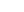times the semi-base (that is, the slope of the face is); the square of the height is equal to the area of a face, φ times the square of the semi-base.

The medial right triangle
Right triangle
A right triangle or right-angled triangle is a triangle in which one angle is a right angle . The relation between the sides and angles of a right triangle is the basis for trigonometry.-Terminology:The side opposite the right angle is called the hypotenuse...

of this "golden" pyramid (see diagram), with sides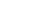is interesting in its own right, demonstrating via the Pythagorean theorem
Pythagorean theorem
In mathematics, the Pythagorean theorem or Pythagoras' theorem is a relation in Euclidean geometry among the three sides of a right triangle...

the relationship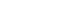or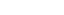. This "Kepler triangle"
is the only right triangle proportion with edge lengths in geometric progression
Geometric progression
In mathematics, a geometric progression, also known as a geometric sequence, is a sequence of numbers where each term after the first is found by multiplying the previous one by a fixed non-zero number called the common ratio. For example, the sequence 2, 6, 18, 54, ... is a geometric progression...

, just as the 3–4–5 triangle is the only right triangle proportion with edge lengths in arithmetic progression
Arithmetic progression
In mathematics, an arithmetic progression or arithmetic sequence is a sequence of numbers such that the difference between the consecutive terms is constant...

. The angle with tangentcorresponds to the angle that the side of the pyramid makes with respect to the ground, 51.827… degrees (51° 49' 38").

A nearly similar pyramid shape, but with rational proportions, is described in the Rhind Mathematical Papyrus
Rhind Mathematical Papyrus
The Rhind Mathematical Papyrus , is named after Alexander Henry Rhind, a Scottish antiquarian, who purchased the papyrus in 1858 in Luxor, Egypt; it was apparently found during illegal excavations in or near the Ramesseum. It dates to around 1650 BC...

(the source of a large part of modern knowledge of ancient Egyptian mathematics
Egyptian mathematics
Egyptian mathematics is the mathematics that was developed and used in Ancient Egypt from ca. 3000 BC to ca. 300 BC.-Overview:Written evidence of the use of mathematics dates back to at least 3000 BC with the ivory labels found at Tomb Uj at Abydos. These labels appear to have been used as tags for...

), based on the 3:4:5 triangle; the face slope corresponding to the angle with tangent 4/3 is 53.13 degrees (53 degrees and 8 minutes). The slant height or apothem is 5/3 or 1.666… times the semi-base. The Rhind papyrus has another pyramid problem as well, again with rational slope (expressed as run over rise). Egyptian mathematics did not include the notion of irrational numbers, and the rational inverse slope (run/rise, multiplied by a factor of 7 to convert to their conventional units of palms per cubit) was used in the building of pyramids.

Another mathematical pyramid with proportions almost identical to the "golden" one is the one with perimeter equal to 2π times the height, or h:b = 4:π. This triangle has a face angle of 51.854° (51°51'), very close to the 51.827° of the Kepler triangle. This pyramid relationship corresponds to the coincidental relationship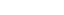.

Egyptian pyramids very close in proportion to these mathematical pyramids are known.

### Egyptian pyramids

In the mid nineteenth century, Röber studied various Egyptian pyramids including Khafre, Menkaure and some of the Giza, Sakkara and Abusir groups, and was interpreted as saying that half the base of the side of the pyramid is the middle mean of the side, forming what other authors identified as the Kepler triangle; many other mathematical theories of the shape of the pyramids have also been explored.

One Egyptian pyramid is remarkably close to a "golden pyramid"—the Great Pyramid of Giza
Great Pyramid of Giza
The Great Pyramid of Giza is the oldest and largest of the three pyramids in the Giza Necropolis bordering what is now El Giza, Egypt. It is the oldest of the Seven Wonders of the Ancient World, and the only one to remain largely intact...

(also known as the Pyramid of Cheops or Khufu). Its slope of 51° 52' is extremely close to the "golden" pyramid inclination of 51° 50' and the π-based pyramid inclination of 51° 51'; other pyramids at Giza (Chephren, 52° 20', and Mycerinus, 50° 47') are also quite close. Whether the relationship to the golden ratio in these pyramids is by design or by accident remains open to speculation. Several other Egyptian pyramids are very close to the rational 3:4:5 shape.

Adding fuel to controversy over the architectural authorship of the Great Pyramid, Eric Temple Bell
Eric Temple Bell
Eric Temple Bell , was a mathematician and science fiction author born in Scotland who lived in the U.S. for most of his life...

, mathematician and historian, claimed in 1950 that Egyptian mathematics would not have supported the ability to calculate the slant height of the pyramids, or the ratio to the height, except in the case of the 3:4:5 pyramid, since the 3:4:5 triangle was the only right triangle known to the Egyptians and they did not know the Pythagorean theorem nor any way to reason about irrationals such as π or φ.

Michael Rice asserts that principal authorities on the history of Egyptian architecture have argued that the Egyptians were well acquainted with the golden ratio and that it is part of mathematics of the Pyramids, citing Giedon (1957). Historians of science have always debated whether the Egyptians had any such knowledge or not, contending rather that its appearance in an Egyptian building is the result of chance.

In 1859, the pyramidologist
Pyramidology
Pyramidology is a term used, sometimes disparagingly, to refer to various pseudoscientific speculations regarding pyramids, most often the Giza Necropolis and the Great Pyramid of Giza in Egypt...

John Taylor claimed that, in the Great Pyramid of Giza
Great Pyramid of Giza
The Great Pyramid of Giza is the oldest and largest of the three pyramids in the Giza Necropolis bordering what is now El Giza, Egypt. It is the oldest of the Seven Wonders of the Ancient World, and the only one to remain largely intact...

, the golden ratio is represented by the ratio of the length of the face (the slope height), inclined at an angle θ to the ground, to half the length of the side of the square base, equivalent to the secant of the angle θ. The above two lengths were about 186.4 and 115.2 meters respectively. The ratio of these lengths is the golden ratio, accurate to more digits than either of the original measurements. Similarly, Howard Vyse
Richard William Howard Vyse
Major-General Sir Richard William Howard Vyse, KCMG was a British soldier, anthropologist and Egyptologist. He was also Member of Parliament for Beverley and Honiton .-Family life:...

, according to Matila Ghyka, reported the great pyramid height 148.2 m, and half-base 116.4 m, yielding 1.6189 for the ratio of slant height to half-base, again more accurate than the data variability.

## Disputed observations

Examples of disputed observations of the golden ratio include the following:
• Historian John Man states that the pages of the Gutenberg Bible
Gutenberg Bible
The Gutenberg Bible was the first major book printed with a movable type printing press, and marked the start of the "Gutenberg Revolution" and the age of the printed book. Widely praised for its high aesthetic and artistic qualities, the book has an iconic status...

were "based on the golden section shape". However, according to Man's own measurements, the ratio of height to width was 1.45.
• Some specific proportions in the bodies of many animals (including humans) and parts of the shells of mollusks and cephalopods are often claimed to be in the golden ratio. There is actually a large variation in the real measures of these elements in specific individuals, and the proportion in question is often significantly different from the golden ratio. The ratio of successive phalangeal bones of the digits and the metacarpal bone has been said to approximate the golden ratio. The nautilus
Nautilus
Nautilus is the common name of marine creatures of cephalopod family Nautilidae, the sole extant family of the superfamily Nautilaceae and of its smaller but near equal suborder, Nautilina. It comprises six living species in two genera, the type of which is the genus Nautilus...

shell, the construction of which proceeds in a logarithmic spiral
Logarithmic spiral
A logarithmic spiral, equiangular spiral or growth spiral is a special kind of spiral curve which often appears in nature. The logarithmic spiral was first described by Descartes and later extensively investigated by Jacob Bernoulli, who called it Spira mirabilis, "the marvelous...

, is often cited, usually with the idea that any logarithmic spiral is related to the golden ratio, but sometimes with the claim that each new chamber is proportioned by the golden ratio relative to the previous one; however, measurements of nautilus shells do not support this claim.
• The proportions of different plant components (numbers of leaves to branches, diameters of geometrical figures inside flowers) are often claimed to show the golden ratio proportion in several species. In practice, there are significant variations between individuals, seasonal variations, and age variations in these species. While the golden ratio may be found in some proportions in some individuals at particular times in their life cycles, there is no consistent ratio in their proportions.
• In investing, some practitioners of technical analysis
Technical analysis
In finance, technical analysis is security analysis discipline for forecasting the direction of prices through the study of past market data, primarily price and volume. Behavioral economics and quantitative analysis incorporate technical analysis, which being an aspect of active management stands...

use the golden ratio to indicate support of a price level, or resistance to price increases, of a stock or commodity; after significant price changes up or down, new support and resistance levels are supposedly found at or near prices related to the starting price via the golden ratio. The use of the golden ratio in investing is also related to more complicated patterns described by Fibonacci numbers; see, e.g. Elliott wave principle
Elliott wave principle
The Elliott Wave Principle is a form of technical analysis that some traders use to analyze financial market cycles and forecast market trends by identifying extremes in investor psychology, highs and lows in prices, and other collective factors...

. See Fibonacci retracement
Fibonacci retracement
In finance, Fibonacci retracements is a method of technical analysis for determining support and resistance levels. They are named after their use of the Fibonacci sequence...

. However, other market analysts have published analyses suggesting that these percentages and patterns are not supported by the data.

• Golden angle
Golden angle
In geometry, the golden angle is the smaller of the two angles created by sectioning the circumference of a circle according to the golden section; that is, into two arcs such that the ratio of the length of the larger arc to the length of the smaller arc is the same as the ratio of the full...

• List of works designed with the golden ratio
• Penrose tiling
Penrose tiling
A Penrose tiling is a non-periodic tiling generated by an aperiodic set of prototiles named after Sir Roger Penrose, who investigated these sets in the 1970s. The aperiodicity of the Penrose prototiles implies that a shifted copy of a Penrose tiling will never match the original...

• Plastic number
Plastic number
In mathematics, the plastic number ρ is a mathematical constant which is the unique real solution of the cubic equationx^3=x+1\, ....

• The Roses of Heliogabalus
The Roses of Heliogabalus
The Roses of Heliogabalus is a famous painting of 1888 by the Anglo-Dutch academician Sir Lawrence Alma-Tadema, at present in private hands, and based on a probably invented episode in the life of the Roman emperor Elagabalus, also known as Heliogabalus, , taken from the Augustan History...

• Sacred geometry
Sacred geometry
Sacred geometry is the geometry used in the planning and construction of religious structures such as churches, temples, mosques, religious monuments, altars, tabernacles; as well as for sacred spaces such as temenoi, sacred groves, village greens and holy wells, and the creation of religious art...

• Silver ratio
Silver ratio
In mathematics, two quantities are in the silver ratio if the ratio between the sum of the smaller plus twice the larger of those quantities and the larger one is the same as the ratio between the larger one and the smaller. This defines the silver ratio as an irrational mathematical constant,...